Question

If the frequency of the motion of a simple harmonic oscillator is doubled, by what factor does the maximum speed of the oscillator change?

1.If the frequency of the motion of a simple harmonic oscillator is doubled , then maximum speed of the oscillator changes by the factor 2

Explanation:

We know that in a simple harmonic oscillator the maximum speed is given by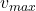=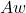Here A is amplitude which is constant , so from above equation we see that maximum speed is directly proportional to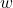of the oscillation .

Since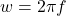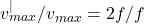= 2

Where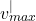is the maximum speed when frequency is doubled .